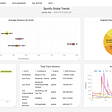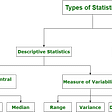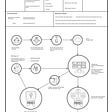# Data Preprocessing

`melted = pd.melt(df, id_vars=['RegionName'], var_name='time')    melted['time'] = pd.to_datetime(melted['time'], infer_datetime_format=True)    melted = melted.dropna(subset=['value'])`

# ARIMA Modeling

`def find_p_and_q(ts, verbose=True):    # Finds the optimal p, d, and q for an arima model    # To start, we create a set of possible pdq combinations    p = range(5)    d = range(3)    q = range(5)    pdq = list(itertools.product(p, d, q))        # Next we run each combination through an arima model and record the AIC    AICs = []    for comb in pdq:        try:            model = ARIMA(ts, order=comb, enforce_stationarity=True, enforce_invertibility=True)            output = model.fit()            AICs.append([comb, output.aic])        except:            # need to have a fail safe in case the arima won't converge            pass    AIC_df = pd.DataFrame(AICs, columns=['pdq', 'AIC'])    if verbose:        # prints the pdq combination with lowest AIC        print(AIC_df.loc[AIC_df.AIC.idxmin()])    # returns the pdq combination with lowest AIC        return AIC_df.loc[AIC_df.AIC.idxmin()]`

# Summary

--

--

--

## More from Chris Grannan

Love podcasts or audiobooks? Learn on the go with our new app.

## Building a Rock Paper Scissors AI## A Year of Ed Sheeran: Analyzing Spotify Global Trends## Newton vs Neural Networks: How AI is Corroding the Fundamental Values of Science.## Python Pandas: A Powerful Tool for Data Analytics## Introduction to Statistics and Its types## Why Data Extraction Services are Better Than Tools for Enterprises## Data Life Cycle Analysis## Significant paper review## Road to Web3 — Wallet Integration## What is an alternative investment?